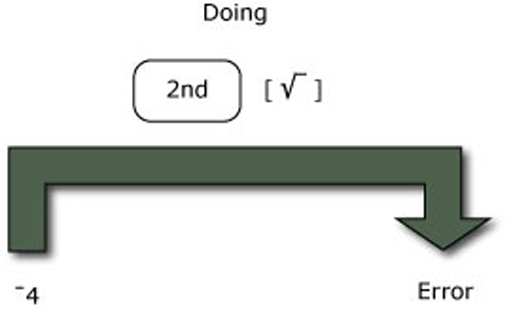Tapping into mathematics

Start this free course now. Just create an account and sign in. Enrol and complete the course for a free statement of participation or digital badge if available.

Free course

# 7.3 Square rooting a negative number

Another problem surfaces if you start with a negative number and try to find its square root. For example try to find the square root of 4 on your calculator. Depending upon how your calculator is set up, you may either get an error message or an unfamiliar number like 2i or 2j. This is because there is no real number which squared will give you the negative number 4. Every real number, whether positive or negative, has a positive square. There are some numbers, called imaginary numbers, which are the square roots of negative numbers and they do have uses in mathematics. You may come across them in more advanced mathematics and some scientific and graphics calculators will allow you to calculate with them. However in general you are likely to get an error message if you try to square root a negative number. So square roots must be handled with care.Figure 8 Finding the square root of a negative number gives an error message
MU120_4MCAL